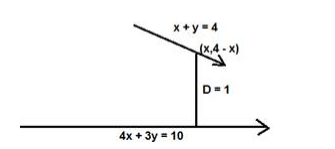# Find all the points on the line x + y = 4 that lie at a unit distance from theQuestion:

Find all the points on the line x + y = 4 that lie at a unit distance from the line 4x+3y=10.

Solution:

Given: points lie on the line x + y = 4 , perpendicular distance = 1 units

To find : points on the line x + y = 4

Formula used: We know that the distance between a point $(m, n)$ and a line $a x+b y+c$ $=0$ is given by,

$D=\frac{|a m+b n+c|}{\sqrt{a^{2}+b^{2}}}$The equation of the line is $4 x+3 y-10=0$ and $D=1$ units

Here $m=x$ and $n=4-x$ (from the equation $x+y=4$ ), $a=4, b=3, c=-10$

$D=\frac{|4(x)+3(4-x)-10|}{\sqrt{4^{2}+3^{2}}}=1$

$D=\frac{|4 x+12-3 x-10|}{\sqrt{16+9}}=\frac{|x-2|}{\sqrt{25}}=\frac{|x-2|}{5}=1$

$|x-2|=1 \times 5=5$

$x-2=5$ or $x-2=-5$

$x=5+2$ or $x=-5+2$

$x=7$ or $x=-3$

We know that the points lie on the line x + y = 4

$y=4-7=-3$ or $y=4-(-3)=7$

$(7,-3)$ and $(-3,7)$ are the points on the line $x+y=4$ that lie at a unit distance from

4x + 3y = 10.